### Home > INT3 > Chapter 2 > Lesson 2.1.1 > Problem2-9

2-9.

For each diagram below, write and solve an equation to calculate the value of the variable. If you need help, refer to the Math Notes box in this lesson. State your answer to part (d) in both approximate decimal form and radical form.

1.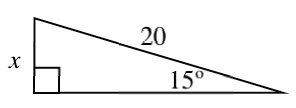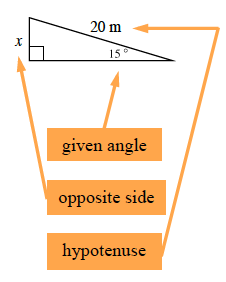$\text{sin}\,\theta=\frac{\text{opposite side}}{\text{hypotenuse}}$

$\text{sin}(15^\circ)=\frac{\textit{x}}{20}$

$20\text{sin}(15^\circ)=20\left(\frac{x}{20}\right)\ \ \ \ \ \ 20\text{sin}(15^\circ)=x$

Use your calculator to compute $x$. Does your answer seem reasonable? If not, verify that your calculator is in degree mode.

1.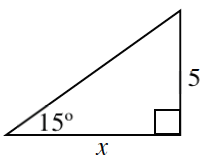$\text{tan}\,\theta=\frac{\text{opposite side}}{\text{adjacent side}}$

Follow the steps in part (a).

1.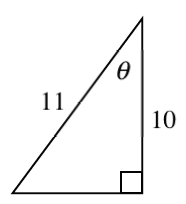$\text{cos}\,\theta=\frac{\text{adjacent side}}{\text{hypotenuse}}$

How can you "undo" cosine?

$\theta=\text{cos}^{-1}\left(\frac{10}{11}\right)\ \ \ \ \theta=24.6^\circ$

1.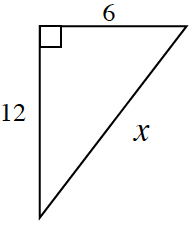An angle is not marked, just three sides. What formula uses three sides of a right triangle?

Pythagorean Theorem